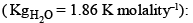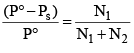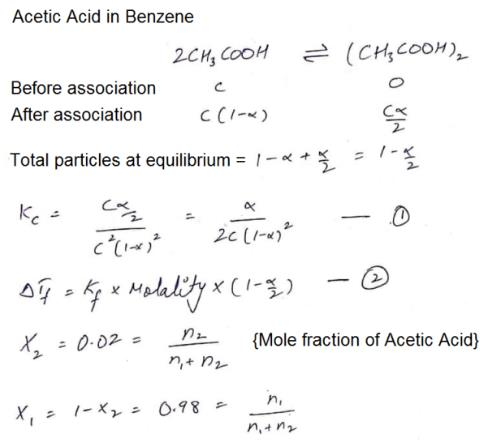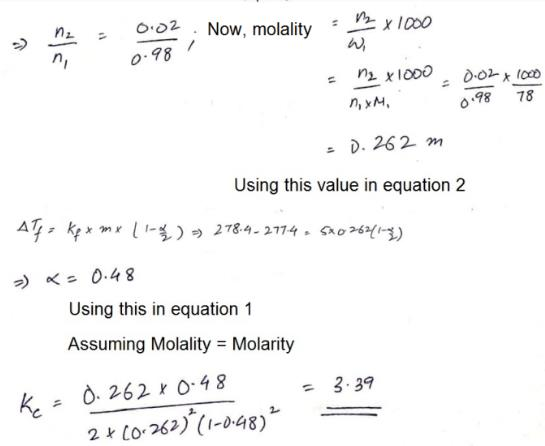Courses

# Test: Colligative Properties - 3

## 25 Questions MCQ Test Physical Chemistry | Test: Colligative Properties - 3

Description
This mock test of Test: Colligative Properties - 3 for Chemistry helps you for every Chemistry entrance exam. This contains 25 Multiple Choice Questions for Chemistry Test: Colligative Properties - 3 (mcq) to study with solutions a complete question bank. The solved questions answers in this Test: Colligative Properties - 3 quiz give you a good mix of easy questions and tough questions. Chemistry students definitely take this Test: Colligative Properties - 3 exercise for a better result in the exam. You can find other Test: Colligative Properties - 3 extra questions, long questions & short questions for Chemistry on EduRev as well by searching above.
QUESTION: 1

Solution:
QUESTION: 2

Solution:
QUESTION: 3

### The vapour pressure of a solvent decreased by 10 mm Hg when a non-volatile solute was added to the solvent. The mole fraction of solute in solution is 0.2, what would be mole fraction of the solvent in decrease in vapour pressure is 20 mm of Hg:

Solution:
QUESTION: 4

Y g of non–volatile organic substance of molecular mass M is dissolved in 250 g benzene. Molal elevation constant of benzene is Kb. Elevation in its boiling point is given by:

Solution:
QUESTION: 5

The freezing point of aqueous solution contains 5% by mass urea, 1.0% by mass KCl and 10% by mass of glucose isSolution:
QUESTION: 6

The values of observed and calculated molecular weights of silver nitrate are 92.64 and 170 respectively. The degree of dissociation of silver nitrate is:

Solution:
QUESTION: 7

Which of the following aqueous solutions has the highest boiling point & minimum freezing point?

Solution:
QUESTION: 8

Among the following the solution which shows the highest osmotic pressure at 25ºC is:

Solution:
QUESTION: 9

If a solute undergoes dimerisation and trimerisation, the minimum values of Vant Hoff factors respectively are:

Solution:
QUESTION: 10

Osmotic pressure of 4% (w/v) urea solution is 1.64 atm and that of 3.42% (w/v) cane sugar is 2.46 atm. When equal volume of the above two solutions are mixed, the osmotic pressure of the resulting solution is:

Solution:
*Multiple options can be correct
QUESTION: 11

When mercuric iodide is added to an aqueous solution of potassium iodide then:

Solution:
*Multiple options can be correct
QUESTION: 12

Which of the following will have same value of Vant Hoff factor (i) as that of K4[Fe(CN)6]:

Solution:
*Multiple options can be correct
QUESTION: 13

If Pº and Ps are the V.P. of solvent and its solution respectively and N1 and N2 are the moles of solute and solvent then:

Solution:

If Po​ and Ps​ are the vapour pressures of the solvent and solution respectively, N1​ and N2 are the mole fractions of the solvent and solute respectively, then Ps= Po​N1​.

According to Raoult's law, the vapour pressure of a solvent above a solution is equal to the vapour pressure of the pure solvent at the same temperature scaled by the mole fraction of the solvent present.*Multiple options can be correct
QUESTION: 14

At constant temperature, the osmotic pressure of a solution is:

Solution:
QUESTION: 15

The value of Ebullioscopic constant or boiling point elevation constant depends on:

Solution:
*Multiple options can be correct
QUESTION: 16

Which of the following pairs of solution are expected to be isotonic at the same temperature:

Solution:
*Multiple options can be correct
QUESTION: 17

Isotonic solution have:

Solution:
*Multiple options can be correct
QUESTION: 18

The Vant Hoff factor is:

Solution:

In case of association of solute particles in solution, the observed molecular weight of solute being more than the normal, the value of factor 'i' is less than unity (i.e. i < 1), while for dissociation the value of i is greater than unity (i.e. i > 1), because the observed molecular weight has lesser value than normal molecular weight.

*Multiple options can be correct
QUESTION: 19

Ideal solution is formed when to components:

Solution:
*Multiple options can be correct
QUESTION: 20

When a non-volatile solute is added to a pure solvent, which statement(s) hold true:

Solution:
*Answer can only contain numeric values
QUESTION: 21

An aqueous solution of glucose boils at 100.01ºC. The mo lal elevat ion constant for water is 0.5 K Kg mol–1. The number of glucose molecules in the solution containing 100 g of water are x × 1021. The value of x is ______.

Solution:
*Answer can only contain numeric values
QUESTION: 22

The apparent degree of ionization of KCl in water at 290 K is 0.86, the mass of KCl which must be made up to 1 dm3 of aqueous solution so as to produce the same osmotic pressure as the 4% solution of glucose at that temperature is ______g.

Solution:
*Answer can only contain numeric values
QUESTION: 23

An aqueous solution containing 288 g of a non–volatile compound having the stoichiometric composit ion CxH2xOx in 90 g water boils at 101.24ºC at 1 atmospheric pressure. What is the value of x? (Given that: Kb(H2O) = 0.512 K Kg mol–1):

Solution:
*Answer can only contain numeric values
QUESTION: 24

The freezing point of a solution of acetic acid (mole fraction is 0.02) in benzene is 277.4 K. Acetic acid exists partly as a dimer. Freezing point of benzene is 278.4 K and Kf for benzene is 5 K kg mol–1. The equilibrium constant for dimerisation is ______ kg mol–1:

Solution:Hence 3.39 is correct

*Answer can only contain numeric values
QUESTION: 25

The relative lowering of vapour pressure of an aqueous solution of a non–volatile solute of molecular weight 60 (which neither dissociates nor associates in the solution) is 0.018. If Kf of water is 1.86º cm–1, the depression in freezing point will be ______ºC.

Solution: